Courses

# Theories Of Column,Theories Of Failure - MCQ Test 1

## 20 Questions MCQ Test Mock Test Series - Mechanical Engineering (ME) for GATE 2020 | Theories Of Column,Theories Of Failure - MCQ Test 1

Description
This mock test of Theories Of Column,Theories Of Failure - MCQ Test 1 for Mechanical Engineering helps you for every Mechanical Engineering entrance exam. This contains 20 Multiple Choice Questions for Mechanical Engineering Theories Of Column,Theories Of Failure - MCQ Test 1 (mcq) to study with solutions a complete question bank. The solved questions answers in this Theories Of Column,Theories Of Failure - MCQ Test 1 quiz give you a good mix of easy questions and tough questions. Mechanical Engineering students definitely take this Theories Of Column,Theories Of Failure - MCQ Test 1 exercise for a better result in the exam. You can find other Theories Of Column,Theories Of Failure - MCQ Test 1 extra questions, long questions & short questions for Mechanical Engineering on EduRev as well by searching above.
QUESTION: 1

### The rod PQ of length L and with flexural rigidity EI is hinged at both ends. For what minimum force F is it expected to buckle?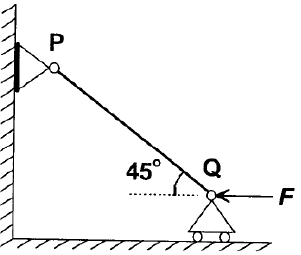Solution:

Axial component of the force FPQ = F Sin 45

We know for both end fixed column buckling load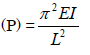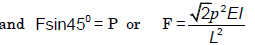QUESTION: 2

Solution:
QUESTION: 3

### The piston rod of diameter 20 mm and length 700 mm in a hydraulic cylinder is subjected to a compressive force of 10 KN due to the internal pressure. The end conditions for the rod may be assumed as guided at the piston end and hinged at the other end. The Young’s modulus is 200 GPa. The factor of safety for the piston rod is

Solution:
QUESTION: 4

A structural member subjected to an axial compressive force is called

Solution:

A machine part subjected to an axial compressive force is called a strut. A strut may
be horizontal, inclined or even vertical. But a vertical strut is known as a column, pillar
or stanchion.
The term column is applied to all such members except those in which failure would be
by simple or pure compression. Columns can be categorized then as:

QUESTION: 5

An axle is a machine part that is subjected to:

Solution:

Axle is a non-rotating member used for supporting rotating wheels etc. and do not transmit any torque. Axle must resist forces applied laterally or transversely to their axes. Such members are called beams.

QUESTION: 6

Mach List-I with List-II and select the correct answer using the codes given below the lists: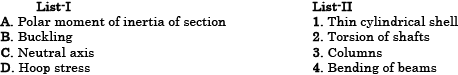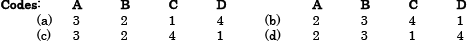Solution:
QUESTION: 7

Assertion (A): A long column of square cross section has greater buckling stability than a similar column of circular cross-section of same length, same material and same area of cross-section with same end conditions.

Reason (R): A circular cross-section has a smaller second moment of area than a square cross-section of same area.

Solution:
QUESTION: 8

Match List-I (End conditions of columns) with List-II (Equivalent length in terms of length of hinged-hinged column) and select the correct answer using the codes given below the Lists: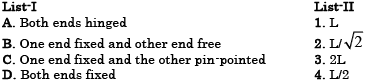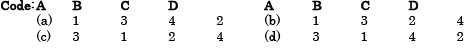Solution:
QUESTION: 9

What is the expression for the crippling load for a column of length „l‟ with one end fixed and other end free?

Solution:
QUESTION: 10

For the case of a slender column of length L and flexural rigidity EI built in at its base and free at the top, the Euler‟s critical buckling load is

Solution:
QUESTION: 11

Euler's formula can be used for obtaining crippling load for a M.S. column with hinged ends.

Which one of the following conditions for the slenderness ratio  1/k is to be satisfied?

Solution:
QUESTION: 12

If one end of a hinged column is made fixed and the other free, how much is the critical load compared to the original value?

Solution: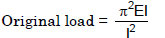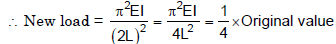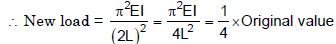QUESTION: 13

The ratio of the compressive critical load for a long column fixed at both the ends and a column with one end fixed and the other end free is:

Solution:

Critical Load for one end fixed, and other end free is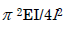and both ends fixed is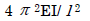QUESTION: 14

A long slender bar having uniform rectangular cross-section 'B x H' is acted upon by an axial compressive force. The sides B and H are parallel to x- and y-axes respectively. The ends of the bar are fixed such that they behave as pin-jointed when the bar buckles in a plane normal to x-axis, and they behave as built-in when the bar buckles in a plane normal to y-axis. If load capacity in either mode of buckling is same, then the value of H/B will be:

Solution: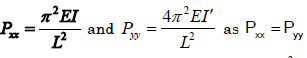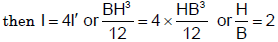QUESTION: 15

If σc  and E denote the crushing stress and Young's modulus for the material of a column, then the Euler formula can be applied for determination of cripping load of a column made of this material only, if its slenderness ratio is:

Solution: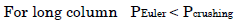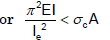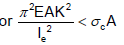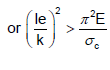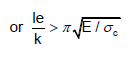QUESTION: 16

Rankine Gordon formula for buckling is valid for

Solution:
QUESTION: 17

Mach List-I with List-II and select the correct answer using the codes given below the lists: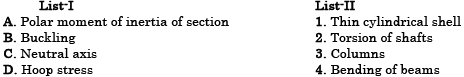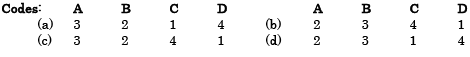Solution:
QUESTION: 18

Which one of the following statements is correct?]

Solution:

A column with both ends fixed has minimum equivalent effective length (l/2)

QUESTION: 19

Assertion (A): Buckling of long columns causes plastic deformation.

Reason (R): In a buckled column, the stresses do not exceed the yield stress.

Solution:

And Critical Load for one end fixed, and other end free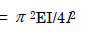QUESTION: 20

Which one of the following pairs is not correctly matched?

Solution:

Buckling factor: The ratio of equivalent length of the column to the least radius of
gyration.